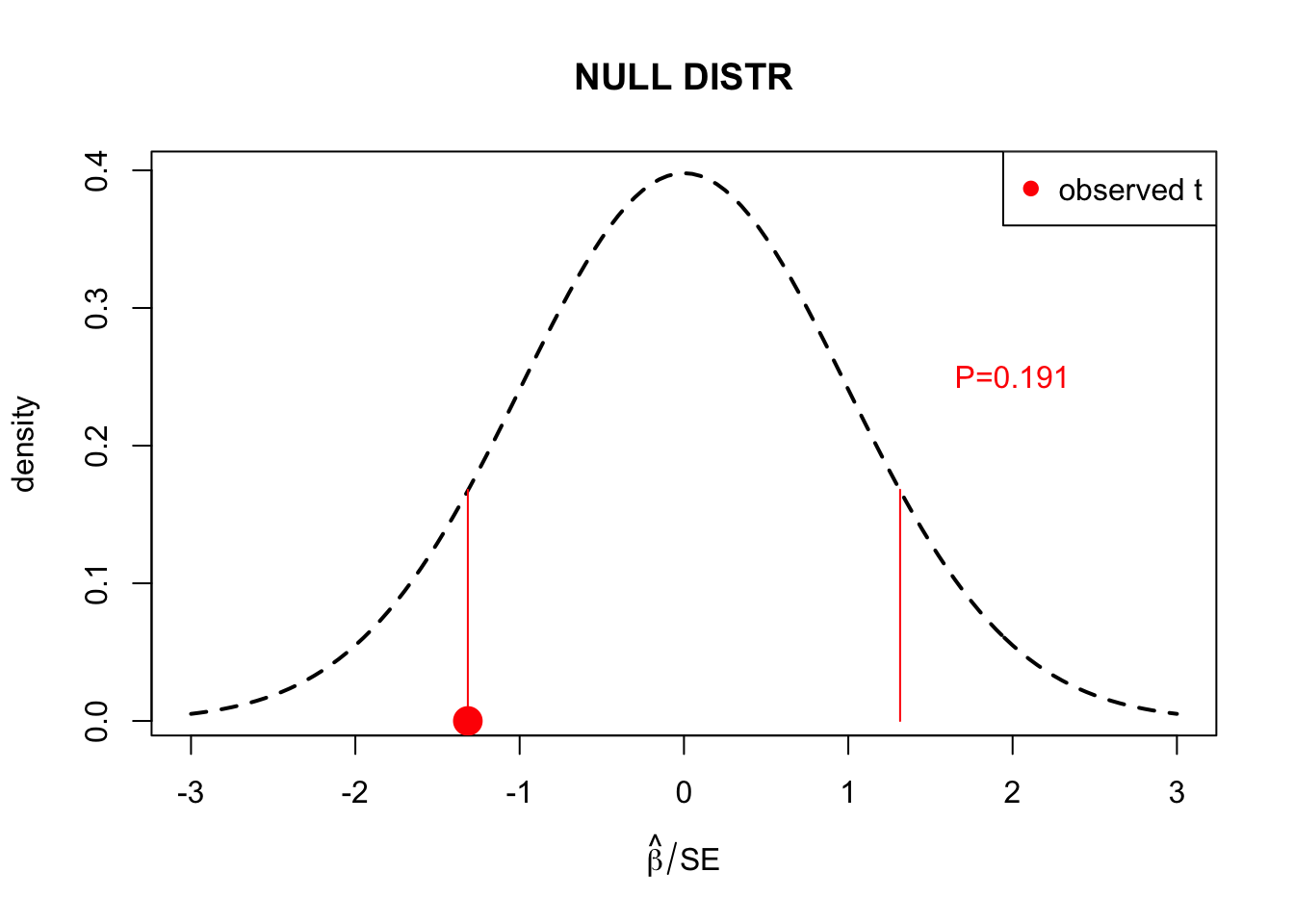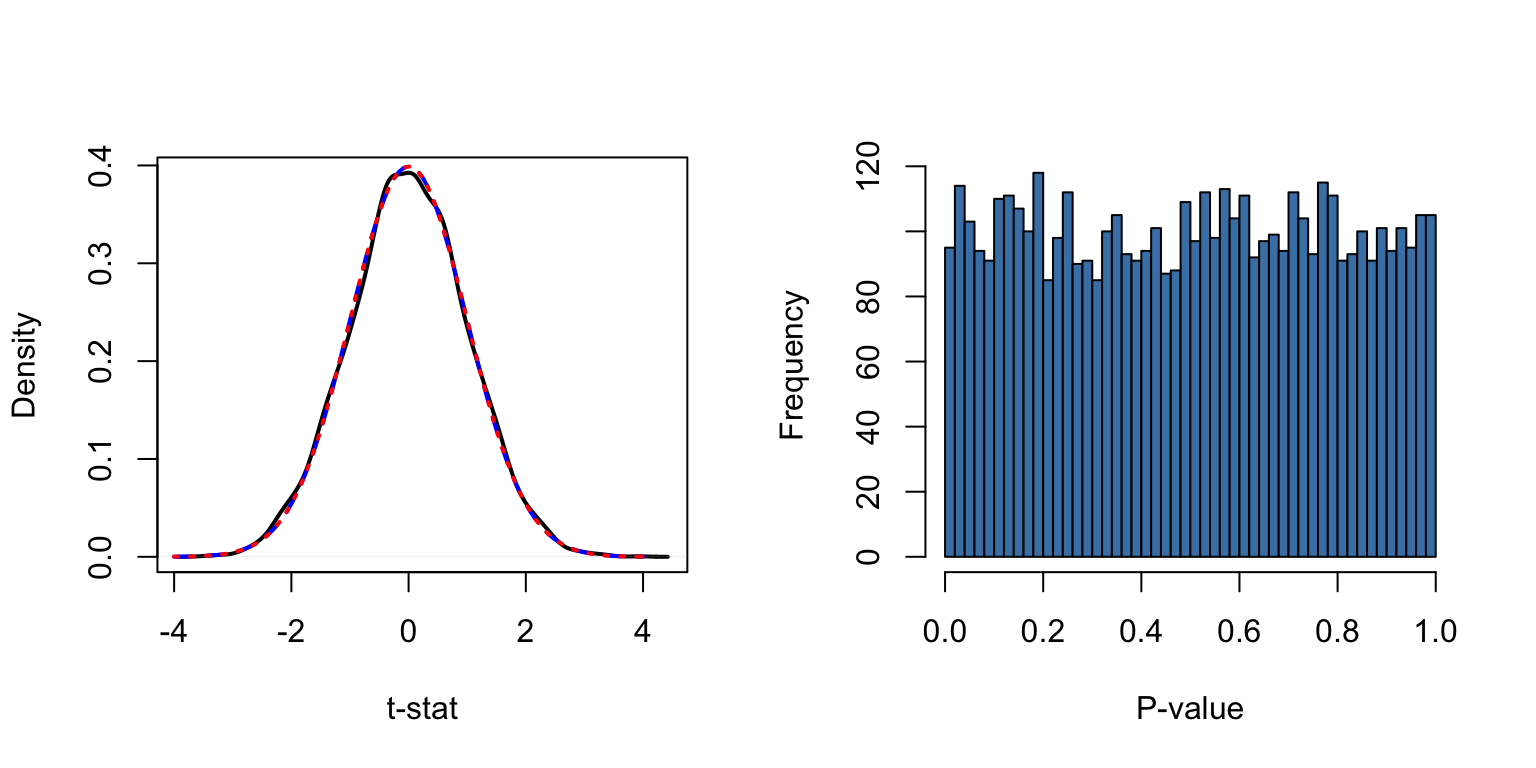Suppose that we have a large number $$p$$ of variables of which some could be explaining outcome $$y$$. How do we know which ones are important? What are the statistical measures we can use to rank the variables?

Example 1.1

1. Genome-wide association study. We have $$n$$ individuals measured on $$p\sim 10^6$$ positions on the genome where each $$x_{ij}$$ has value of 0,1 or 2 denoting how many copies of the reference DNA letter the individual $$i$$ carries at position $$j$$ of the genome. In addition, each individual has been measured for cholesterol levels (outcome $$y$$). Which genomic positions affect cholesterol levels? We can do linear regression of $$y$$ on each predictor $$x_j$$ separately which leads to the regression summary statistics ($$\widehat{\beta_j}$$,$$\textrm{SE}_j$$,$$P_j$$) for each $$x_j$$. The task is to infer which of the $$10^6$$ predictors are truly altering cholesterol levels.

2. Tumour - normal comparison. We compare the levels of $$p$$ (in thousands) proteins between the tumour sample and a control sample from a healthy tissue of the same patient. When we have hundreds of patients we can compute t-statistics from a paired t-tests for each protein to see whether it has different levels in tumour than in healthy tissue across the patients. Again we end up with $$p$$ estimates for differences ($$\widehat{\beta}$$), their SEs and P-values, one for each of the $$p$$ proteins. Which proteins are statistically clearly differentiated between tumour and normal samples?

3. Brain images. Imaging data are very high dimensional. For example, we could define thousands of regions from the brain that could be compared between certain groups of individuals, (e.g. groups stratified by age, sex or a psychiatric condition). How do we determine which regions show differential activity between the groups?

P-value

In a standard statistical inference framework, P-value is used as a basis for inference. The purpose for using P-value is to see whether the observed data seem inconsistent with the null hypothesis. Typically, the null hypothesis states that the variable is not important, or technically, that its effect size is 0. We have one null hypothesis per each variable.

P-value is a probability of getting something “at least as extreme” as what has been observed, if the null hypothesis was true.

Therefore, a small P-value is interpreted as evidence that the null hypothesis may not be true. Logic goes that if P-value is very small, then it would be very unlikely to observe the kind of data at hand under the null hypothesis – and therefore either the null hypothesis is not true or we have encountered a very unlikely event.

A more formal definition of P-value

Suppose that we have

• observed data $$y$$,
• defined a null hypothesis (NULL) that determines a way to generate data sets that are similarly structured as $$y$$,
• defined a test statistic $$t = t(Y)$$ whose value can be computed from the data in such a way that larger values of the test statistic (in our opinion) imply higher discrepancy between data and the null hypothesis.

P-value (of data $$y$$) is a probability that if additional data $$Z$$ were generated according to the null hypothesis, then the corresponding test statistic $$t(Z)$$ computed from data $$Z$$ would be at least as large as our observed $$t(y)$$. That is, $\textrm{P-value of data y is } \textrm{Pr}(t(Z) \geq t(y)\, |\, Z \sim \textrm{NULL}).$ Important. P-value is NOT a probability that the null hypothesis is true: P-value is probability of certain kind of data under the null hypothesis, it is NOT a probability of the null hypothesis given data.

Example 1.2

Let’s do one linear regression with $$p=1$$ and put its P-value in its place in the null distribution of t-statistic. The goal is to test whether the slope ($$\beta_1$$) of the model $$y=\beta_0 + x \beta_1 + \varepsilon$$ is zero. The null hypothesis is $$H_0: \beta_1 =0$$. By fitting the linear model with lm() we get the estimate $$\widehat{\beta}_1$$ of slope and its P-value. Here P-value tells that if the true slope $$\beta_1=0$$, what is the probability that we observe a data set from which the computed slope is at least as large (in absolute value) as our observed $$\widehat{\beta}_1$$. Most often we don’t look at the null distribution of $$\widehat{\beta}_1$$, which depends on sample size and variances of $$x$$ and $$\varepsilon$$, but instead we look at the null distribution of the t-statistics $$t=\widehat{\beta}_1/\textrm{SE}_1$$ which has distribution $$t(n-p-1)$$, i.e., t-distribution with $$n-p-1$$ degrees of freedom. (When $$n-p-1>50$$, $$t(n-p-1)$$ is very accurately the same as $$\mathcal{N}(0,1)$$ and hence doesn’t noticably depend on the sample size.)

set.seed(29)
n = 100
x = rnorm(n) # predictor
y = rnorm(n) # outcome, independent of x
lm.fit = lm(y ~ x)
x.grid = seq(-3, 3, 0.05) # to define the plotting region
plot(x.grid, dt(x.grid, df = n-2), lty = 2, lwd = 2, t = "l",
xlab = expression(hat(beta)/SE), ylab = "density", main = "NULL DISTR") #null distribution of t-stat.
t.stat = summary(lm.fit)$coeff[2,3] # observed t-statistic: Estimate/SE points(t.stat, 0, pch = 19, cex = 2, col = "red") segments(t.stat*c(1,-1), c(0, 0), t.stat*c(1, -1), rep(dnorm(t.stat, 0, 1),2), col = "red") text(2, 0.25, paste0("P=",signif(summary(lm.fit)$coeff[2,4], 3)), col = "red")
legend("topright", pch = 19, col = "red", leg = "observed t")P-value is the probability mass outside the red segments, i.e., the sum of the two tail probabilities. It tells how probable, under the null, it is to get at least as extreme observation as we have got here, when the extremeness is measured as distance away from 0.

GAME: Guess a P-value

What is (approximately) the P-value

1. That in 10 fair coin tosses we get 9 Heads and 1 Tails? Is it $$10^{-2}$$ or $$10^{-7}$$ or $$10^{-17}$$?

2. That in 100 fair coin tosses we get 90 Heads and 10 Tails? Is it $$10^{-2}$$ or $$10^{-7}$$ or $$10^{-17}$$?

3. That if we (say, 20 people) would make a random sitting order, you would keep your current place? Is it $$0.05$$ or $$10^{-3}$$ or $$10^{-20}$$?

4. That if we (say, 20 people) would make a random sitting order, no-one would change places? Is it $$0.05$$ or $$10^{-3}$$ or $$10^{-20}$$?

5. That physicists used as significance level to claim Higg’s Boson found in 2012? Is it $$0.05$$ or $$10^{-4}$$ or $$10^{-7}$$?

S-value

P-value is often used as an attempt to quantify how “surprised” one is when observing a particular kind of data set assuming that the null hypothesis holds. However, without experience, it may not be easy to interpret how surprised one would be, for example when observing a P-value of, say, 0.03 or 0.001. S-value (or surprise value) is a tool for giving a more intuitive interpretation to numerical P-values.

Think that you are flipping $$n$$ coins and your null hypothesis is that the coins are fair, that is, have probability of 0.5 of landing heads up. Suppose that you observed that all coins landed heads up. This is not at all surprising if you only have one or two coins since such events happen for a fair coin with probability of 0.5 ($$n=1$$) or 0.25 ($$n=2$$). However, if you have $$n=100$$ coins and all land heads up, then it seems almost impossible to believe that the coins are fair since the observed outcome seems so surprising under the null hypothesis.

For any $$n$$, the probability (under the null) of observing only heads in $$n$$ tosses is $$2^{-n}$$. We can use this to turn any observed probability $$P$$ (such as P-value) to a corresponding $$n = -\log_2(P)$$ that tells that how many coins should we have observed to have yielded only heads in order for us to experience the same amount of surprise as what observing an event with probability $$P$$ describes. This value $$n$$ is called S-value corresponding to the given probability $$P$$.

Thus, for example, the standard P-value threshold of 0.05 corresponds to S-value of $$n(0.05)=-\log_2(0.05)=4.32$$ coin flips, P-value of 0.001 corresponds to $$n(0.001)=-\log_2(0.001)=9.97$$ coin flips and P-value of $$10^{-6}$$ corresponds to $$n(10^{-6})=-\log_2(10^{-6})=19.93$$ coin flips.

Liberal P-value threhsolds and replicability crisis

Recently, there has been much discussion about how traditional use of P-values (“significance testing at threshold of P < 0.05”) has led to poor replicability of the reported scientific findings and hence poor science, in particularly with high dimensional data sets with “multiple testing issues”. We will have a look at this problem next. For this discussion, see also

Distribution of summary statistics

Let’s generate some data, first without any real effects. Our purpose is to see how a large set of P-values behave.

set.seed(6102017)
n = 1000 #individuals
p = 5000 #variables measured on each individual
X = matrix( rnorm(n*p), n, p) #just random variables
y = rnorm(n) #outcome variable that is not associated with any of x

#by mean-centering y and each x, we can ignore intercept terms (since they are 0, see Lecture 0)
X = as.matrix( scale(X, scale = F) ) #mean-centers columns of X to have mean 0
y = as.vector( scale(y, scale = F) )

#apply lm to each column of X separately and without intercept (see Lecture 0.)
lm.res = apply(X, 2 , function(x) summary(lm(y ~ -1 + x))\$coeff[1,])
# lm.res has 4 rows: beta, SE, t-stat and P-value
pval = lm.res[4,] #pick P-values

par(mfrow = c(1,2))
plot(density(lm.res[3,]), sub = "", xlab = "t-stat", main = "", lwd = 2) #should be t with n-2 df
curve(dt(x, df = n-2), from = -4, to = 4, add = T, col = "blue", lwd = 2, lty = 2) #t distr in blue
curve(dnorm(x, 0, 1), from = -4, to = 4, add = T, col = "red", lwd = 2, lty = 3)#normal distr in red
hist(pval, breaks = 50, xlab = "P-value", main = "", col = "steelblue")On left we see that the empirical distribution of t-statistic (black) accurately follows its theoretical $$t(n-2)$$ distribution (blue), and that since $$n$$ is large enough, this distributions is indistinguishable from the normal distribution $$\mathcal{N}(0,1)$$ (red).

Histogram on right shows that the P-values seem distributed uniformly between 0 and 1. This is indeed their distribution when the data follows the null hypothesis, as we will establish later. (To quantitatively assess whether the histogram truly looks “uniform”, we can determine that under the unifrom distribution we would expect each bin to have $$p/50 = 5000/50 = 100$$ P-values and that with $$\geq 95$$% probability, in any one bin, the value would be within interval

qbinom(c(0.025 ,0.975), size = p, prob = 1/50)
##   81 120

Thus, the variation in the histogram seems consistent with the null distribution.)

Let’s also compare the distributions via QQ-plots. First t-statistics against the normal distribution and then P-values against the uniform distribution. To see particularly well the smallest P-values, which are often the most interesting, we will show P-values on -log10 scale.

par(mfrow = c(1, 2)) #Let's make qqplots for t-stats and for P-values
qqnorm(lm.res[3,], cex = 0.5, pch = 3)
qqline(lm.res[3,], col = "red")
#((1:p)-0.5) / p gives us
#p equally spaced values in (0,1) to represent quantiles of Uniform(0,1).
qqplot(-log10( ((1:p)-0.5) / p), -log10(pval), xlab = "Theoretical",
ylab = "Observed", main = "QQ-plot for -log10 P-val", cex = 0.5, pch = 3)
abline(0, 1, col = "red")